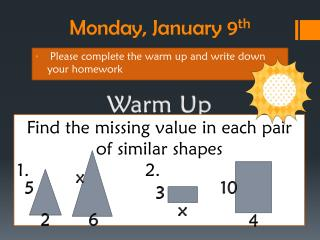DownloadDownload PresentationMonday, January 9 th

# Monday, January 9 th

Télécharger la présentation## Monday, January 9 th

- - - - - - - - - - - - - - - - - - - - - - - - - - - E N D - - - - - - - - - - - - - - - - - - - - - - - - - - -
##### Presentation Transcript

1. Warm Up Monday, January 9th Find the missing value in each pair of similar shapes 1. 2. x 5 10 3 Please complete the warm up and write down your homework x 2 6 4

2. Corny Joke of the Day Why did the teacher have to wear sunglasses to school? Her students were too BRIGHT

3. What? Checkpoint #1 Similar figures Indirect measurement When? TODAYYYYYYYY

4. Week Schedule • Monday: Scale Drawings NOTES HW Scale Drawing Worksheet/Weekly Review • Tuesday: Class PROJECT HW STUDY GUIDE • Wednesday: Revie HW STUDY • Thursday: Test HW NONE • Friday: WEEKLY REVIEW DUE, Test Corrections, Translations NOTES HW NONE

5. Weekly Review Due Friday! Remember to show your work on a separate sheet of paper 

6. A and B are similar. Find y B. What Did Tiggerdo wrong? A. 14 7 4 x

7. Flashcards Indirect measurement

8. What is It? Indirect Measurement uses similar figures and proportions to find height of objects you cannot measure directly

9. Checkpoint • Please put your name on it • Make sure to READ each question carefully • Be sure to show your work • Put in front bin when finished • Complete Weekly Review

10. Attention! Pezzino Travel Company needs your help! They need some expert map readers to find distances between famous landmarks

11. First you must learn how to interpret scale drawings!

12. I Spy

13. Buzz word #1 The map shown is a scale drawing. A scale drawingis a drawing of a real object that is proportionally smaller or larger than the real object. In other words, measurements on a scale drawing are in proportion to the measurements of the real object.

14. Buzz word #2 A scale is a ratio between two sets of measurements. In the map above, the scale is 1 in:100 mi. This ratio means that 1 inch on the map represents 100 miles.

15. Setting it up Set up a proportion: ratio equals ratio Ratio #1 is the scale factor they give you (first 2 numbers) Ratio #2 place third number according to measurement Measurements match in numerators Measurements math in denominators

16. Examples

17. Is it set up right?Why or why not? 1. The scale on the map is 3 cm: 10 m. On the map the distance between two cities is 40 cm. What is the actual distance? 2. The scale on the map is 10 in: 50. On the map the distance between two schools is 30 in. What is the actual distance?

18. Problem #1 Let’s Try Together!

19. 20 in. 4 in. 4x 20 _____ ____ ___ ___ 1 mi 4 4 x mi The scale on a map is 4 in: 1 mi. On the map, the distance between two towns is 20 in. What is the actual distance? Write a proportion using the scale. Let x be the actual number of miles between the two towns. = 1 • 20= 4 •x The cross products are equal. 20= 4x x is multiplied by 4. = Divide both sides by 4 to undo multiplication. 5 = x 5 miles

20. Problem #2 Let’s Try Together!

21. 18 in. 3x 3 in. 18 ___ ___ ____ _____ 1 mi 3 x mi 3 The scale on a map is 3 in: 1 mi. On the map, the distance between two cities is 18 in. What is the actual distance? Write a proportion using the scale. Let x be the actual number of miles between the two cities. = 1 • 18= 3 •x The cross products are equal. 18= 3x x is multiplied by 3. = Divide both sides by 3 to undo multiplication. 6 = x 6 miles

22. Problem #3 You Try!

23. The scale on a map is 2 in: 50 mi. On the map, the distance between two lakes is 12 in. What is the actual distance?

24. Problem #4 Astronomy

25. 18.3 in. 1 in. _________ ___________ x million km 30 million km If a drawing of the planets was made using the scale 1 in:30 million km, the distance from Mars to Jupiter on the drawing would be about 18.3 in. What is the actual distance between Mars to Jupiter? Write a proportion. Let x be the actual distance from Mars to Jupiter. = The cross products are equal. 30 • 18.3= 1 •x 549 = x The actual distance from Mars to Jupiter is about 549 million km.

26. Problem #5 Rafting

27. Our class decides to go white-water rafting on the river. The map we have of the river has a scale of 3 inches equals 10 miles. On the map, the distance between their start point and end point is 18 inches. What is the actual distance in miles? Write a proportion. Let x be the actual distance between start to end Solve for x

28. Can you Answer the EQ? Double time 2 minutes to answer it 2 minutes to share with elbow partner 2 minute discuss with class